# Solution June 22, 2007

### Problem

Does there exist a a sequence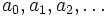$a_{0},a_{1},a_{2},\dots$in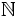$\mathbb N$, such that for each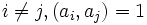$i\neq j, (a_{i},a_{j})=1$, and for each n, the polynomial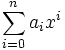$\sum_{i=0}^{n}a_{i}x^{i}$is irreducible in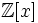$\mathbb Z[x]$?

### Solution

Yes.

Lemma: Let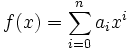$f(x) = \sum_{i=0}^{n}a_ix^i$ with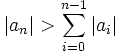$|a_n| > \sum_{i=0}^{n-1}|a_i|$. Then, any complex root of f(x) has absolute value less than 1.

Proof: Assume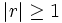$|r|\geq 1$. Then, by the triangle inequality,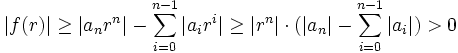$|f(r)| \geq |a_n r^n| - \sum_{i=0}^{n-1}|a_ir^i| \geq |r^n|\cdot(|a_n| - \sum_{i=0}^{n-1}|a_i|) > 0$

so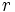$r\,$ is not a root of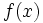$f(x)\,$. The Lemma follows.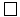$\Box$

Now define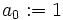$a_0 := 1\,$ and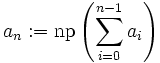$a_n := \mathrm{np}\left(\sum_{i=0}^{n-1}a_i\right)$ where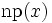$\mathrm{np}(x)\,$ denotes the smallest prime number larger than$x\,$. This sequence satisfies the conditions of the problem.

Proof: Assume there is a n such that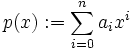$p(x) := \sum_{i=0}^n a_ix^i$ is reducible, i.e.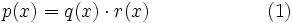$p(x) = q(x)\cdot r(x)\qquad\qquad\qquad(1)\,$

with non-constant polynomials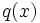$q(x)\,$ and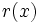$r(x)\,$. Because the leading coefficient of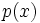$p(x)\,$ is prime, one of the polynomials$q(x)\,$ and$r(x)\,$ must be monic (have leading coefficient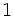$1\,$). Assume without loss of generality that$q(x)\,$ is monic.

By the Fundamentel Theorem of Algebra (http://en.wikipedia.org/wiki/Fundamental_theorem_of_algebra) we can write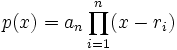$p(x) = a_n \prod_{i=1}^n (x-r_i)$

where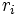$r_i\,$ are the complex roots of$p(x)\,$. By our lemma,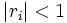$|r_i|<1\,$ for all$i\,$.

All roots of$q(x)\,$ are also roots of$p(x)\,$. The constant term of$q(x)\,$ is the product of these roots. The absolute value of that product is less than$1\,$, so the constant term of$q(x)\,$ - being an integer - must be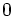$0\,$. But then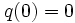$q(0)=0\,$, a contradidiction because 0 is not a root of$p(x)\,$ - indeed,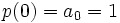$p(0) = a_0 = 1\,$.

So$p(x)\,$ must be irreducible as claimed.$\Box$